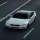Home
IT Knowledge
Inspiration
Languages
EN

# PostgreSQL - calculate average of column values and display the result with no decimals

0 points
Created by:Brian-Tompset
401

In this article, we would like to show you how to calculate the average of column values and display the result with no decimals PostgreSQL.

Quick solution:

``````SELECT ROUND(AVG("column_name"), 0)
FROM "table_name";``````

## Practical example

To show how to calculate the average of column values and display the result with no decimals, we will use the following table:

Note:

At the end of this article you can find database preparation SQL queries.

### Example

In this example, we will display the average salary rounded to 0 decimal places with `ROUND()` function.

Query:

``````SELECT ROUND(AVG("salary"), 0)
FROM "users";``````

Output:

Note:

Go to this article to read more about `ROUND()` function - PostgreSQL - ROUND() function example.

## Database preparation

`create_tables.sql` file:

``````CREATE TABLE "users" (
"id" SERIAL,
"name" VARCHAR(50) NOT NULL,
"surname" VARCHAR(50) NOT NULL,
"department_id" INTEGER,
"salary" DECIMAL(15,2) NOT NULL,
PRIMARY KEY ("id")
);``````

`insert_data.sql` file:

``````INSERT INTO "users"
( "name", "surname", "department_id", "salary")
VALUES
('John', 'Stewart', 1, '3512.00'),
('Chris', 'Brown', 2, '1344.00'),
('Kate', 'Lewis', 3, '6574.00'),
('Ailisa', 'Gomez', NULL, '6500.00'),
('Gwendolyn', 'James', 2, '4200.00'),
('Simon', 'Collins', 4, '3320.00'),
('Taylor', 'Martin', 2, '1500.00'),
('Andrew', 'Thompson', NULL, '2100.00');``````